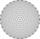# Triangle Problems

#### Number of problems found: 1067

• Sphere cutsAt what distance from the center intersects sphere with radius R = 56 plane, if the cut area and area of the main sphere circle is in ratio 1/2.
• TangentsTo circle with a radius of 41 cm from the point R guided two tangents. The distance of both points of contact is 16 cm. Calculate the distance from point R and circle centre.
• Triangle anglesThe angles α, β, γ in triangle ABC are in the ratio 6:2:6. Calculate size of angles.
• RT - hypotenuse and altitudeRight triangle BTG has hypotenuse g=117 m and altitude to g is 54 m. How long are hypotenuse segments?
• TrianglesEquilateral triangle with side 40 cm has the same perimeter as an isosceles triangle with arm of 45 cm. Calculate the base x of an isosceles triangle.
• Triangle SAAThe triangle has one side long 71 m and its two internal angles is 60°. Calculate the perimeter and area of the triangle.
• SteepleSteeple seen from the road at an angle of 75°. When we zoom out to 25 meters, it is seen at an angle of 20°. What is high?
• Right triangleLegs of the right triangle are in the ratio a:b = 2:8. The hypotenuse has a length of 87 cm. Calculate the perimeter and area of the triangle.
• Heron backlawCalculate missing side in a triangle with sides 17 and 34 and area 275.Ladder 8 m long is leaning against the wall. Its foot is 1 m away from the wall. In which height ladder touches the wall?
• Circle chordWhat is the length x of the chord circle of diameter 115 m, if the distance from the center circle is 11 m?
• Cube diagonalDetermine the length of the cube diagonal with edge 37 mm.
• Isosceles right triangleCalculate the area of an isosceles right triangle whose perimeter is 377 cm.
• Square and circlesSquare with sides 83 cm is circumscribed and inscribed with circles. Determine the radiuses of both circles.
• Triangle ABCRight triangle ABC with right angle at the C, |BC|=18, |AB|=33. Calculate the height of the triangle hAB to the side AB.
• Triangle SASCalculate the area and perimeter of the triangle, if the two sides are 51 cm and 110 cm long and angle them clamped is 130 °.
• N-gon anglesWhat is the sum of interior angles 8-gon? What is the internal angle of a regular convex 8-polygon?
• Square diagonalCalculate the length of the square diagonal if the perimeter is 476 cm.
• Triangle SSSCalculate perimeter and area of ​​a triangle ABC, if a=53, b=46 and c=40.Passenger car and an ambulance come to the rectangular crossroad, the ambulance left. Passenger car at speed 39 km/h and ambulance at 41 km/h. Calculate such a relative speed of the ambulance move to the car.